## Constants of the Motion for a Free Particle

We know that operators representing constants of the motion commute with the Hamiltonian. The form of the Dirac equation we have been using does not have a clear Hamiltonian. This is true essentially because of the covariant form we have been using. For a Hamiltonian formulation, we need to separate the space and time derivatives. Lets find the Hamiltonian in the Dirac equation.Its easy to see the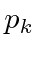commutes with the Hamiltonian for a free particle so that momentum will be conserved.

The components of orbital angular momentum do not commute with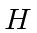.The components of spin also do not commute with.However, the helicity, or spin along the direction of motion does commute.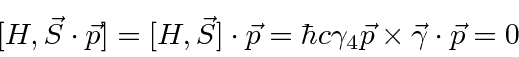From the above commutatorsand, the components of total angular momentum do commute with.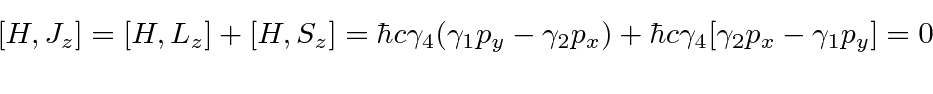The Dirac equation naturally conserves total angular momentum but not conserve the orbital or spin parts of it.

We will need another conserved quantity for the solution to the Hydrogen atom; something akin to the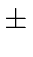inwe used in the NR solution. We can show that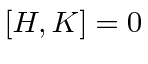forIt is related to the spin component along the total angular momentum direction. Lets compute the commutator recalling thatcommutes with the total angular momentum.It is also useful to show that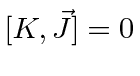so that we have a mutually commuting set of operators to define our eigenstates.This will be zero ifand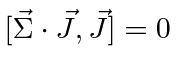.So for the Hydrogen atom,,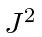,, andform a complete set of mutually commuting operators for a system with four coordinates,,and electron spin.

Jim Branson 2013-04-22